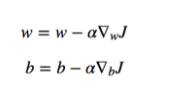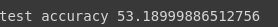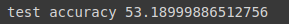Submit a blog to KDnuggets -- Top Blogs Win A Reward

Topics: AI | Data Science | Data Visualization | Deep Learning | Machine Learning | NLP | Python | R | Statistics

KDnuggets Home » News » 2021 » Feb » Tutorials, Overviews » Saving and loading models in TensorFlow — why it is important and how to do it ( 21:n06 )

# Saving and loading models in TensorFlow — why it is important and how to do it

So much time and effort can go into training your machine learning models. But, shut down the notebook or system, and all those trained weights and more vanish with the memory flush. Saving your models to maximize reusability is key for efficient productivity.Photo by Nana Smirnova on Unsplash.

In this article, we are going to discuss the following topics

• Importance of saving deep learning models (in general, not limited to TensorFlow).
• How to save deep learning models in TensorFlow 2, and different types, categories, and techniques of saving the models.
• Loading the saved models in TensorFlow 2.

### Importance of saving deep learning models

Remember, in gradient descent, we update the weights and bias based on the error or loss function.Now imagine you trained a model for thousands of epochs for days or weeks or even hours, and get pretty good weights for your model, meaning that your model is performing a lot well, and then you lose all the weights when you close your program/jupyter notebook.

This will turn into a more hectic problem when you want to reuse that model in another application, and you have no saved progress. You have to start the training process from scratch, which might waste your hours or days.

Practically you can imagine a scenario that you have coded a really good facial recognition model application with above 99% accuracy, precision, etc., and it took you around 30 hours to train that model on a big dataset. Now, if you have not saved the model, and you want to use it in any application, you would have to retrain the whole model for 30 hours.

This is why saving the model is a very important step and can save you a ton of time and resources with just some extra lines of code.

### Saving models in TensorFlow 2

There are 2 different formats to save the model weights in TensorFlow. The first one is the TensorFlow native format, and the second one is the hdf5 format, also known as h5 or HDF format.

Also, there are 2 different ways of saving models.

• Simple, and less complex way, but gives you no freedom.
• Using callbacksto save the model that allows you a lot of freedom, such as saving per-epoch, saving after every n number of examples etc.

We will discuss both in detail.

Let’s load important python libraries and dataset first.

```import tensorflow as tf
from tensorflow.keras.models import Sequential
from tensorflow.keras.layers import Dense, Flatten, Conv2D, MaxPooling2D
(x_train, y_train), (x_test, y_test) = tf.keras.datasets.cifar10.load_data() #cifar10 dataset
x_train = x_train / 255.0 #normalizing images
x_test = x_test / 255.0

```

### Simple Way

The simple way to save the model in TensorFlow is that we can use the built-in function of Tensorflow.Keras.models “Model saving & serialization APIs” that is the save_weights method.

Let’s say we have a sequential model in TensorFlow.

```model = Sequential([
Conv2D(filters=16, input_shape=(32, 32, 3), kernel_size=(3, 3), activation='relu', name='conv_1'),
MaxPooling2D(pool_size=(4, 4), name='pool_1'),
tf.keras.layers.BatchNormalization(),
Flatten(name='flatten'),
Dense(units=32, activation='relu', name='dense_1'),
tf.keras.layers.Dropout(0.5),
Dense(units=10, activation='softmax', name='dense_2')
])
model.compile(optimizer='adam',
loss='sparse_categorical_crossentropy',
metrics=['accuracy'])

```

And now we fit the model using model.fit function in TensorFlow.

```hist = model.fit(x_train,y_train,epochs=5, batch_size=512)

```

We can evaluate the performance of our model via,

```loss, acc = model.evaluate(x_test, y_test, verbose=0)
print(f"test accuracy {acc*100}")

```Now we can save our model just by calling model.save function and passing in the filepath as the argument. This will save the model’s

• Model Architecture
• Model Weights
• Model optimizer state (so that you can continue the training from where you left)
```model.save('myModel.h5')

```

Now adding the extension is important. If you add .h5 as the extension, it will save the model as hdf5 format, and if no extension is provided, the model is saved as TensorFlow native format.

Now when the model is saved in the current directory as myModel.h5 file, you can simply load it in a new program, or same program as a different model via,

```new_model = tf.keras.models.load_model('my_model.h5') #same file path

```

We can check the accuracy of the new loaded model via,

```loss, acc = new_model.evaluate(x_test, y_test, verbose=0)
print(f"test accuracy {acc*100}")

```And we can see that we are getting exactly the same accuracy as the old model.

We can confirm it further by checking the model summary.

```newmodel.summary()

```

And the new summary is precisely identical to our original model’s summary.

```Model: "sequential"
_________________________________________________________________
Layer (type)                 Output Shape              Param #
=================================================================
conv_1 (Conv2D)              (None, 30, 30, 16)        448
_________________________________________________________________
pool_1 (MaxPooling2D)        (None, 7, 7, 16)          0
_________________________________________________________________
batch_normalization (BatchNo (None, 7, 7, 16)          64
_________________________________________________________________
flatten (Flatten)            (None, 784)               0
_________________________________________________________________
dense_1 (Dense)              (None, 32)                25120
_________________________________________________________________
dropout (Dropout)            (None, 32)                0
_________________________________________________________________
dense_2 (Dense)              (None, 10)                330
=================================================================
Total params: 25,962
Trainable params: 25,930
Non-trainable params: 32
_________________________________________________________________

```

Similarly, we can save the weights in TensorFlow native format via,

```new_model.save('newmodel')

```

See how we have not added any file format after the name. This will save our model in TensorFlow native format in the folder newmodel. If we peak into the folder, then we can check what the files are with

```!dir newmodel

```

This command will only run in the jupyter notebook, so alternatively, you can open the folder and check the files.

You will always have 1 file and 2 folders that are:

• assets (folder)
• pb
• variables (folder)

We will have a look at what these folders and files are later. But simply to load the model, we just have to give the pathname which we used to save the model, such as with

```other_model = tf.keras.models.load_model('newmodel')

```

And you can confirm that it is the same model simply via checking its summary or evaluating it to match the results.

Now to save the weights only using the simple way, you just have to call the built-in function save_weights on your model.

Let's take the same old model,

```model = Sequential([
Conv2D(filters=16, input_shape=(32, 32, 3), kernel_size=(3, 3), activation='relu', name='conv_1'),
MaxPooling2D(pool_size=(4, 4), name='pool_1'),
tf.keras.layers.BatchNormalization(),
Flatten(name='flatten'),
Dense(units=32, activation='relu', name='dense_1'),
tf.keras.layers.Dropout(0.5),
Dense(units=10, activation='softmax', name='dense_2')
])
model.compile(optimizer='adam',
loss='sparse_categorical_crossentropy',
metrics=['accuracy'])

```

and train it for a few epochs.

```model.fit(x_train,y_train,epochs=5, batch_size=512)

```

Now you can simply save the weights via,

```path = 'weights_folder/my_weights'
model.save_weights(path)

```

This will create a folder named weights_folder and save the weights in Tensorflow native format with the name of my_weights. It is going to have 3 files.

• checkpoint
• data-00000-of-00001
• index

Let's take a look at these files.

1. my_weights.index

This file tells TensorFlow which weights are stored where. When running models on distributed systems, there may be different shards, meaning the full model may have to be recomposed from multiple sources. In the last notebook, you created a single model on a single machine, so there is only one shard, and all weights are stored in the same place.

1. my_weights.data-00000-of-00001

This file contains the actual weights from the model. It is by far the largest of the 3 files. Recall that the model you trained had around 14000 parameters, meaning this file is roughly 12 bytes per saved weight.

1. checkpoint

This file is by far the smallest. It’s actually so small that we can just look at it directly. It’s a human-readable file with the following text,

```model_checkpoint_path: "my_weights"
all_model_checkpoint_paths: "my_weights"

```

Now when you have saved the weights, you can simply load them by just calling,

```model.load_weights(path)

```

This will load the weights for that model at that specific path.

Alternatively, you can save the weights only in the hdf5 format via,

```model.save_weights('my_weights.h5')

```

This will create a my_weights.h5 file in your working directory, and you can simply load them via model.load_weights('my_weights.h5').

### Important Point

When you are loading the weights for a model, you need to have the correct architecture of that model.

For example:

You can not load the weights of our model we just created to a sequential model with 1 Dense layer, as both are not compatible. So you might be thinking, what is the use of saving the weights only?

Well, the answer is that if you are looking at some big SOTA application, such as YOLO, or something like that where they give you the source code. But, to train them on your machines is a long and lengthy task, so they also give you the pre-trained weights on different epochs, such as if you want to see how this model is performing at 50 epochs, then you can load the saved weights of 50 epochs, and similarly for other numbers of epochs. In this way, you can check the performance of the model on the number of training epochs based on how the model is performing on X number of epochs without explicitly training it.

### TensorFlow Native format vs. hdf5, which to use and when

You have seen that using the .h5 format is simple and clean as it only creates one single file, whereas using tensorflow native format creates multiple folders and files, which is difficult to read. So, you might be thinking that why should we use tensorflow native format? The answer to this is that in the TensorFlow native format, everything is structural and organized in its place. For example, the .pb file contains structural data that can be loaded by multiple languages. Some of the advantages of TF native format are listed in the following.

Advantages of the TensorFlow native format

• TensorFlow’s Servinguses it when you want to take your model to production.
• Language-agnostic — binary format can be read by multiple languages (Java, Python, Objective-C, and C++, among others).
• Advised to use since 0, you can see the official serializing guideof TensorFlow, which recommends using TensorFlow Native format.
• Saves various metadata of the model such as optimizer information, losses, learning rate, etc., which can help later.

Disadvantages

• SavedModelis conceptually harder to grasp than a single file
• Creates a separate folder to store the weights.

Advantages of h5

• Used to save giant data, which might not be tabular.
• Common file saving format.
• Everything saved in one file (weights, losses, optimizers used with keras)

Disadvantages

• Cannot be used with Tensorflow Servingbut you can simply convert it to .pb via experimental.export_saved_model(model, 'path_to_saved_model')

What to use

If you are not going to use TensorFlow while serving or deploying your model, then for simplicity, you can use .hdf5 format, but if you are going to use TensorFlow serving, then you should use tensorflow native format.

### Learning Outcome

In this article, you learned

• Why should you save your machine learning model.
• How to save model weights only using the simple method.
• How to save a complete model using the simple method.
• Saving in TensorFlow Native format or HDF5 format.
• Difference between TensorFlow Native and HDF5 format and what to use.

For more details, check out:

Related:

## Top Stories Past 30 Days

Most PopularGet KDnuggets, a leading newsletter on AI, Data Science, and Machine Learning

By subscribing you accept KDnuggets Privacy Policy